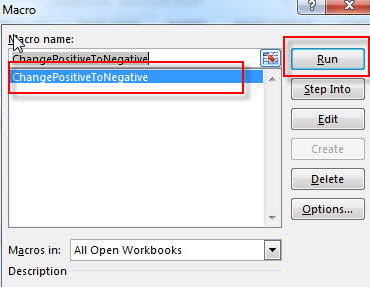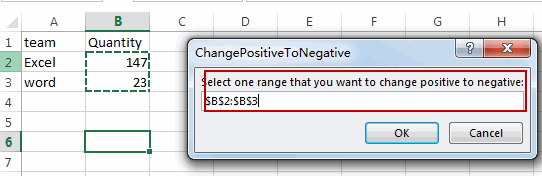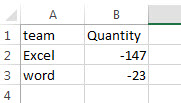# How to Convert Positive Numbers to Negative

This tutorial will teach you how to change positive numbers to negative with Paste special multiply operation in excel. How to convert positive number to negative or how to reverse the number signs with VBA code in Excel.

## Convert Positive Numbers to Negative with Paste Special Operation

To change positive numbers to negative in excel, you can do it with copy and paste, you need to copy a cell that contains a negative number -1, then select the cells that contain the positive numbers, and use the multiply operation on the Pasted Special menu. Then it will perform the multiplication on the selected cell. So all positive number will be change to negative.

You can do it as following steps:

1# type one negative number –1 in one cell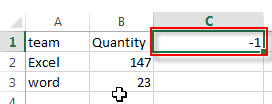2# select that cell that contains -1 and then copy it.

3# select the range of cells that contain positive numbers, then right-click on it, select Paste Special from the pop-up menu list.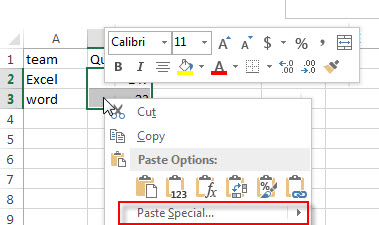4# the Paste Special window will appear.

5# select Multiply radio button from the Operation section.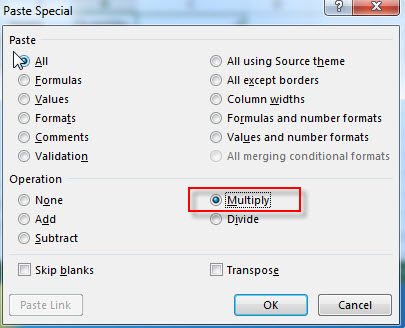6# Click OK button, you will see that all of the positive numbers should be change to negative.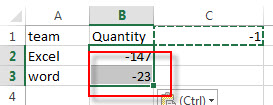If the selected range of cells contains negative numbers, then its values also will be multiplied by -1, so its signs also will be reversed as negative.

You can also write an Excel formula to multiply one negative number -1 to achieve the same result. the generic formula is like this:

`=B1* -1`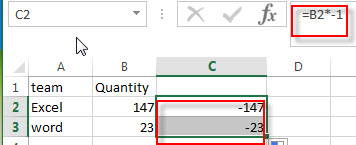## Convert Positive Numbers to Negative with VBA Code

You can also write a new excel macro to convert positive numbers to negative numbers in Excel VBA, just do the following:

1# click on “Visual Basic” command under DEVELOPER Tab.2# then the “Visual Basic Editor” window will appear.

3# click “Insert” ->”Module” to create a new module4# paste the below VBA code into the code window. Then clicking “Save” button.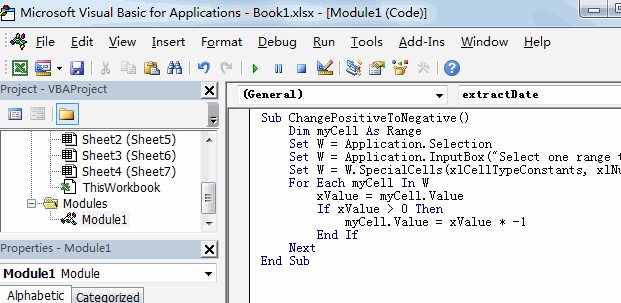```Sub ChangePositiveToNegative()
Dim myCell As Range
Set W = Application.Selection
Set W = Application.InputBox("Select one range that you want to change positive to negative:", "ChangePositiveToNegative", W.Address, Type:=8)
Set W = W.SpecialCells(xlCellTypeConstants, xlNumbers)
For Each myCell In W
xValue = myCell.Value
If xValue > 0 Then
myCell.Value = xValue * -1
End If
Next
End Sub```

5# back to the current worksheet, then run the above excel macro.